Ex 5.1

Chapter 5 Class 10 Arithmetic Progressions (Term 2)
Serial order wise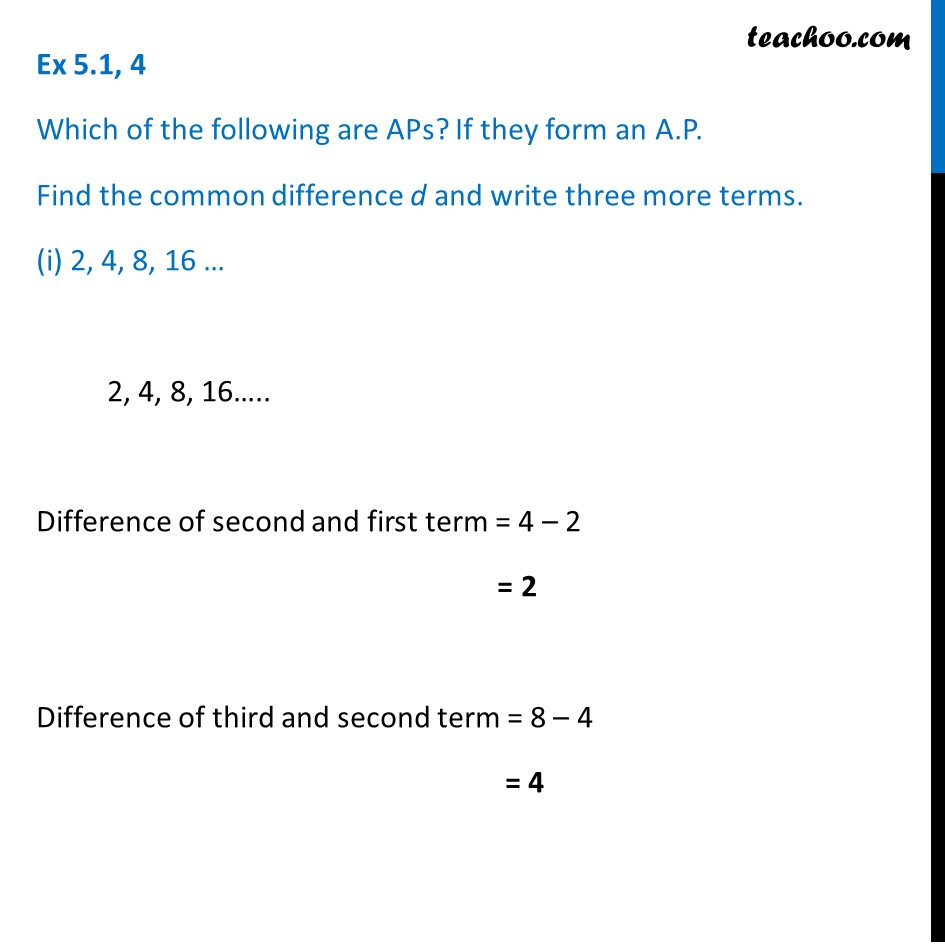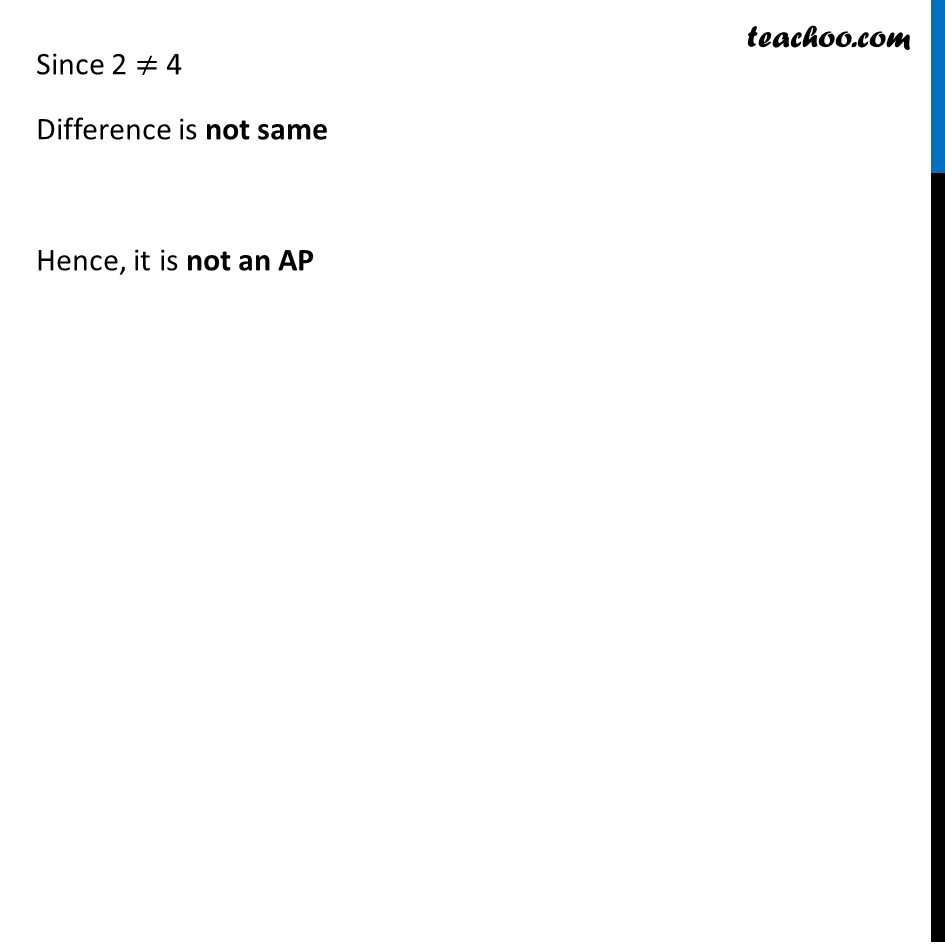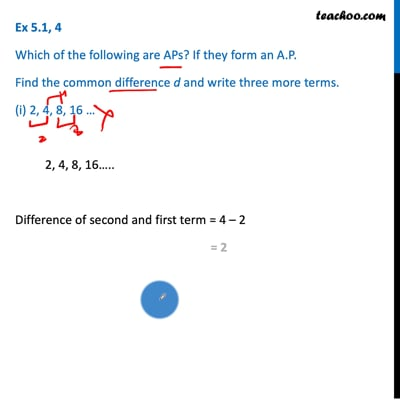This video is only available for Teachoo black users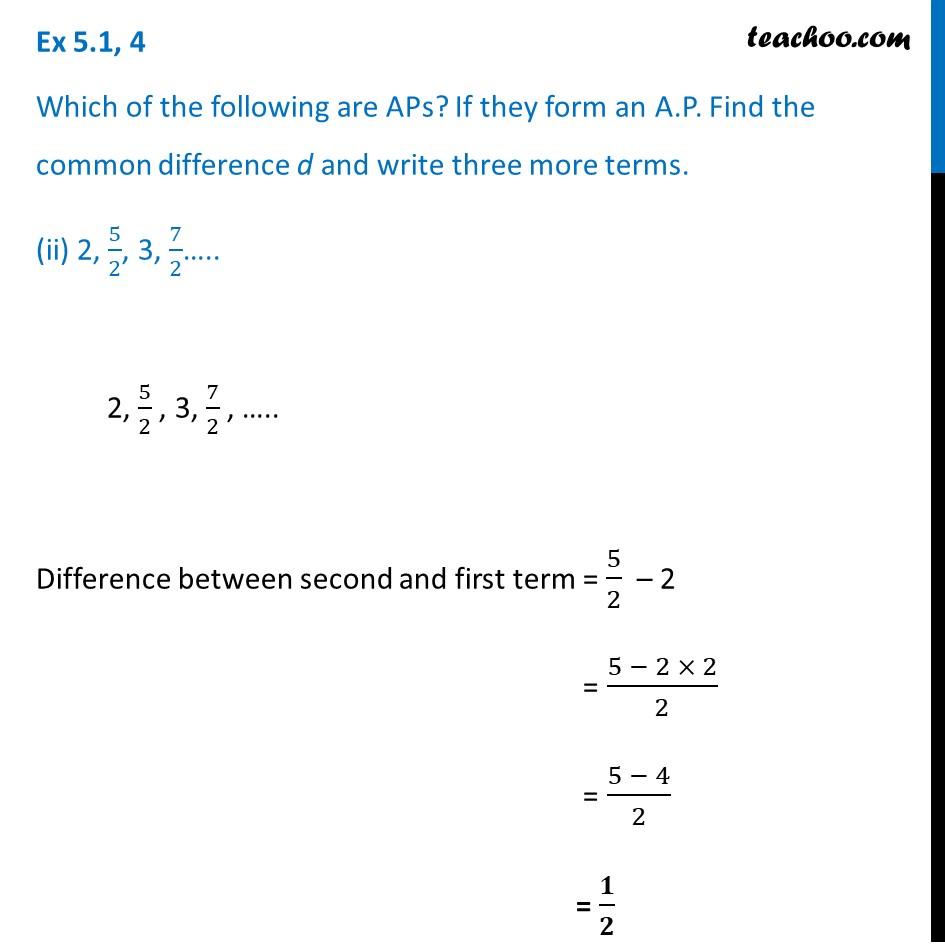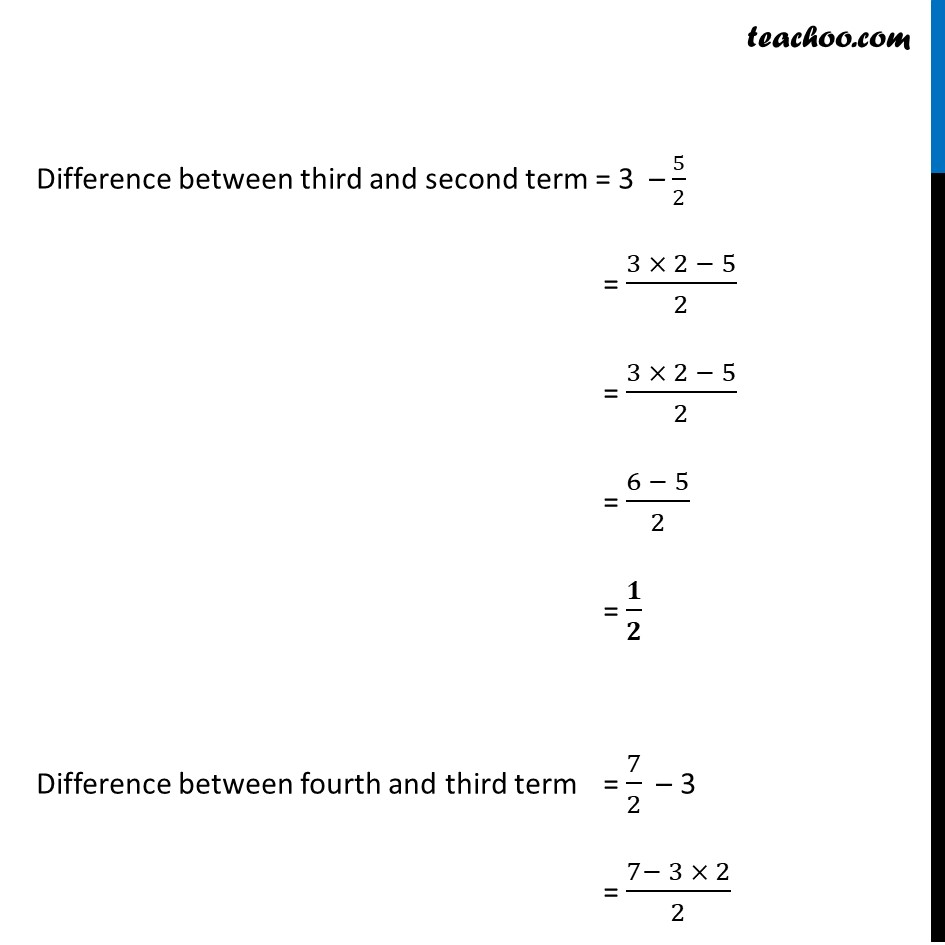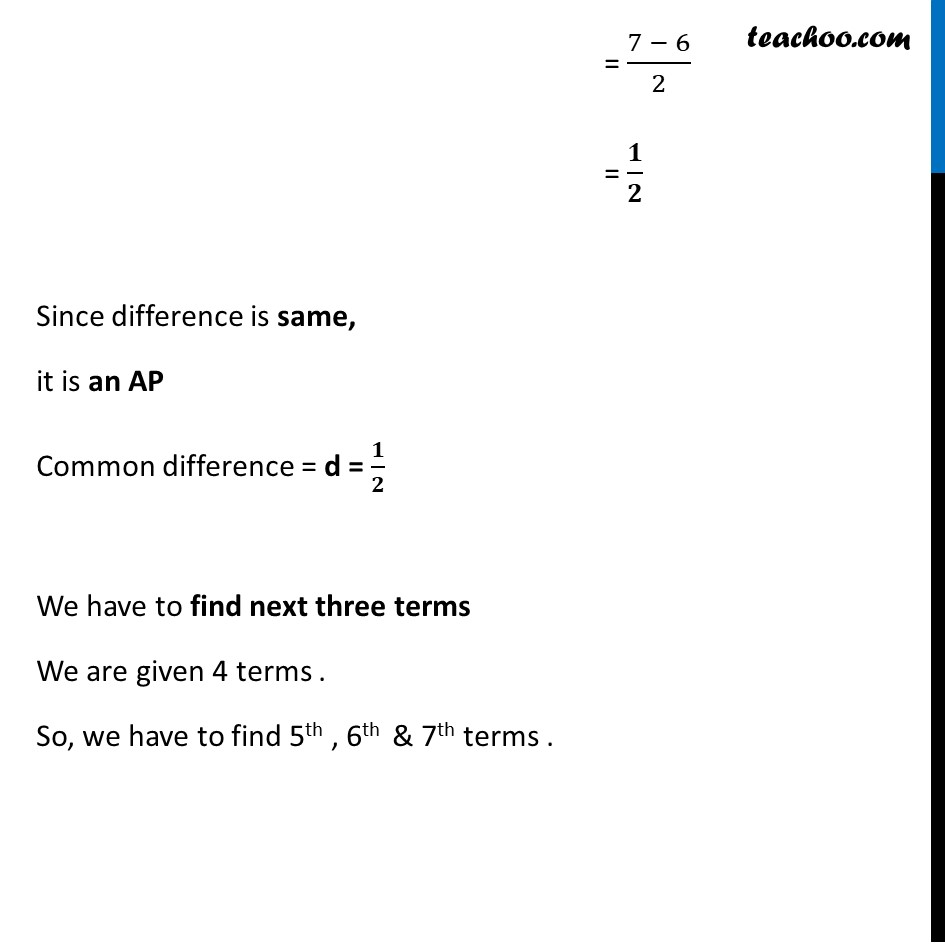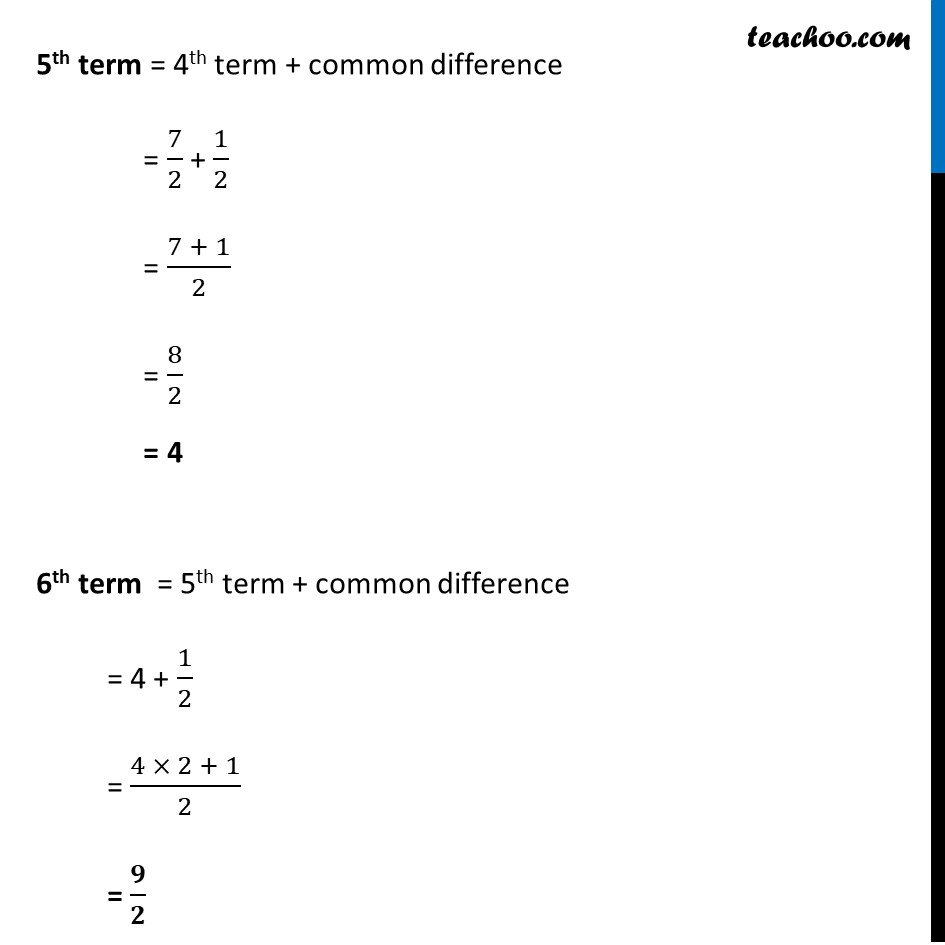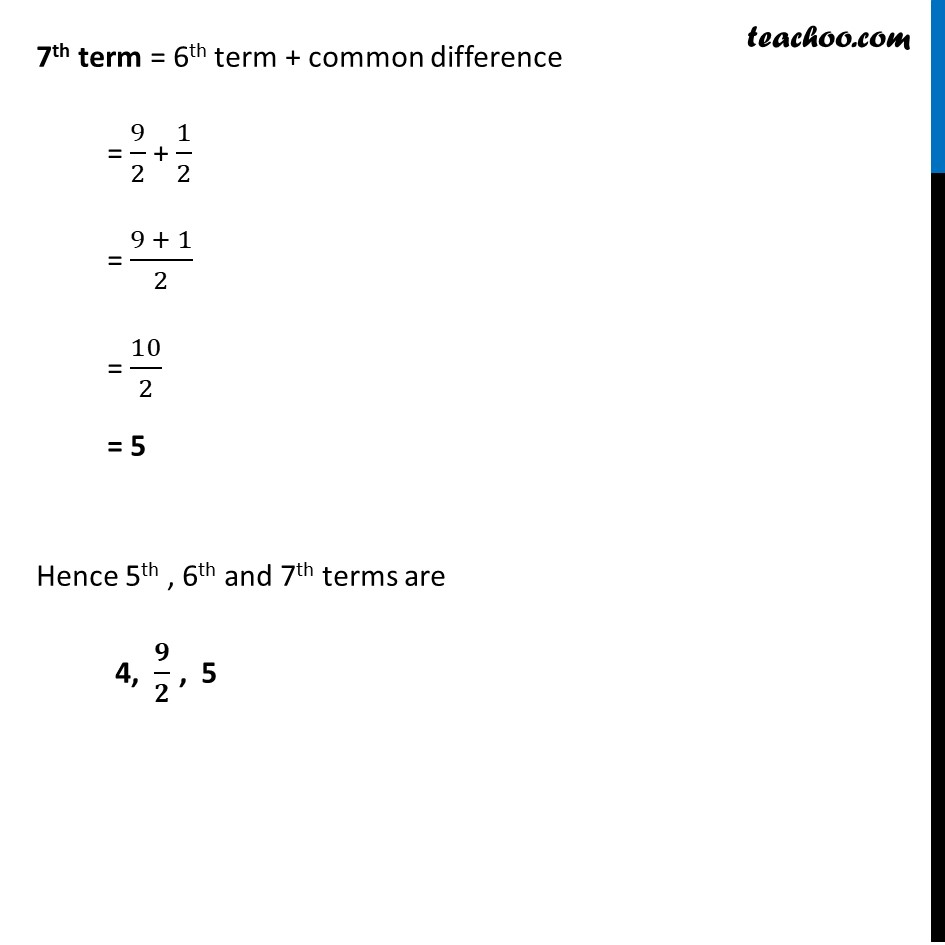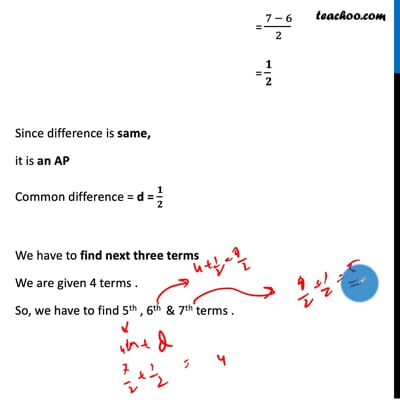This video is only available for Teachoo black users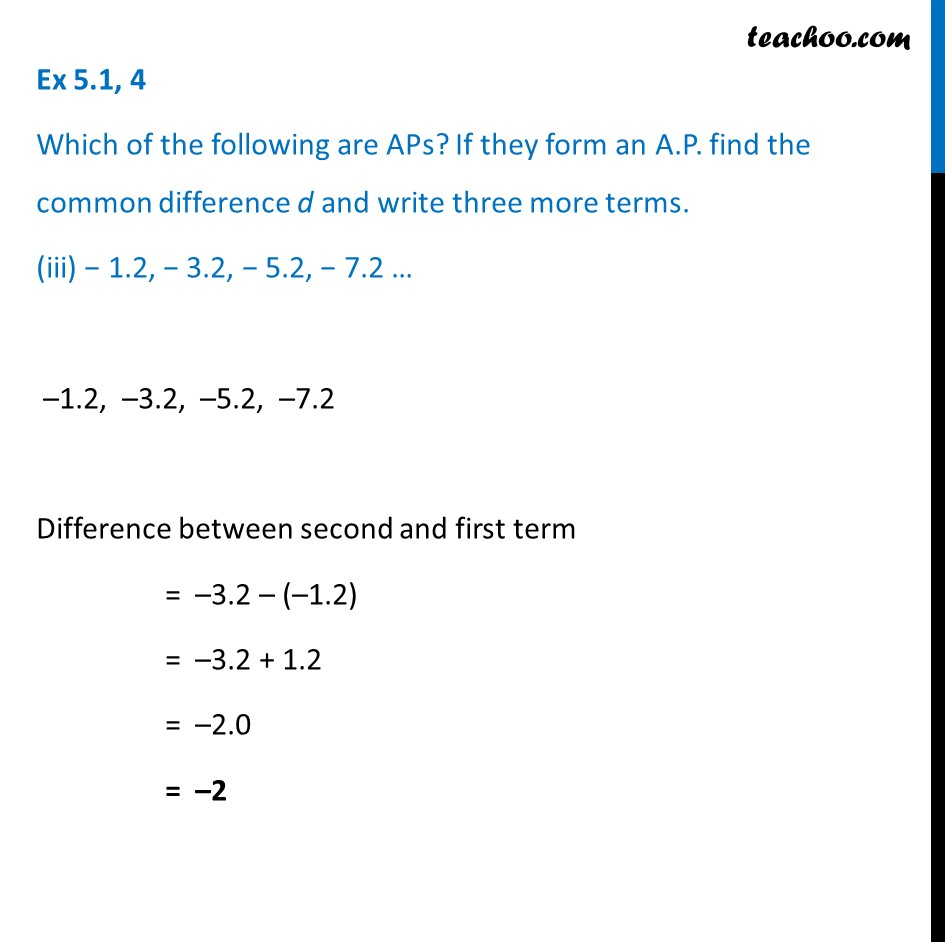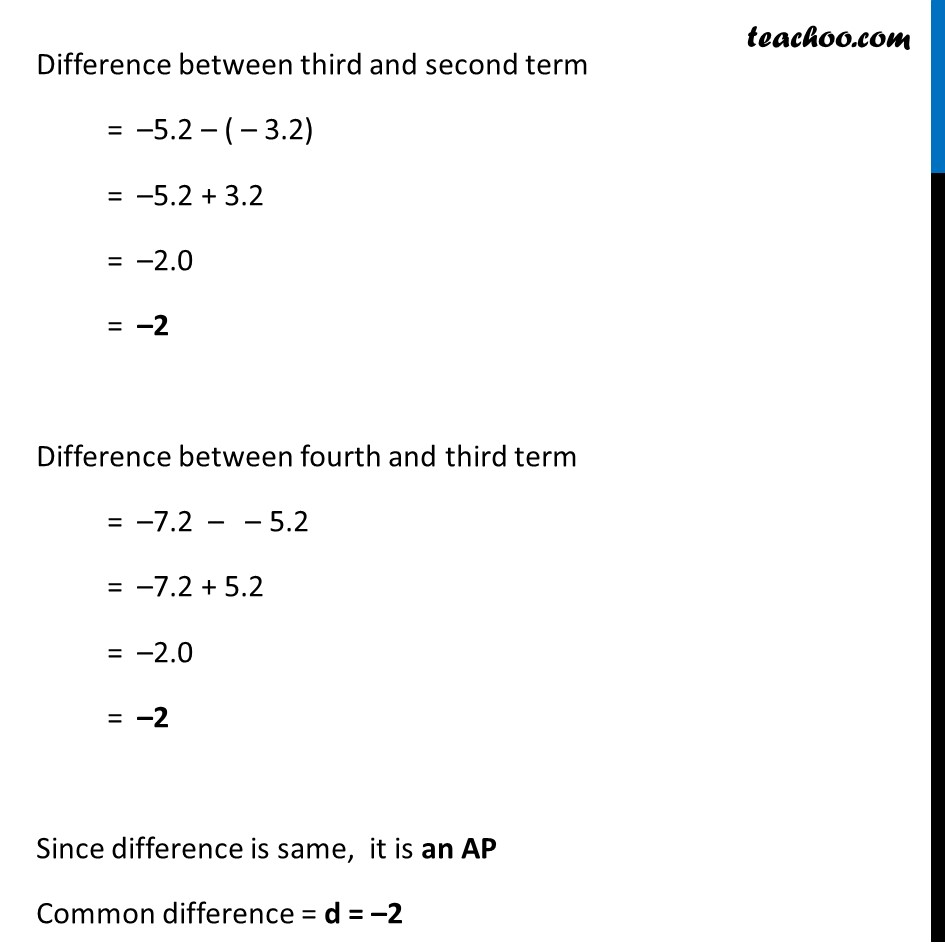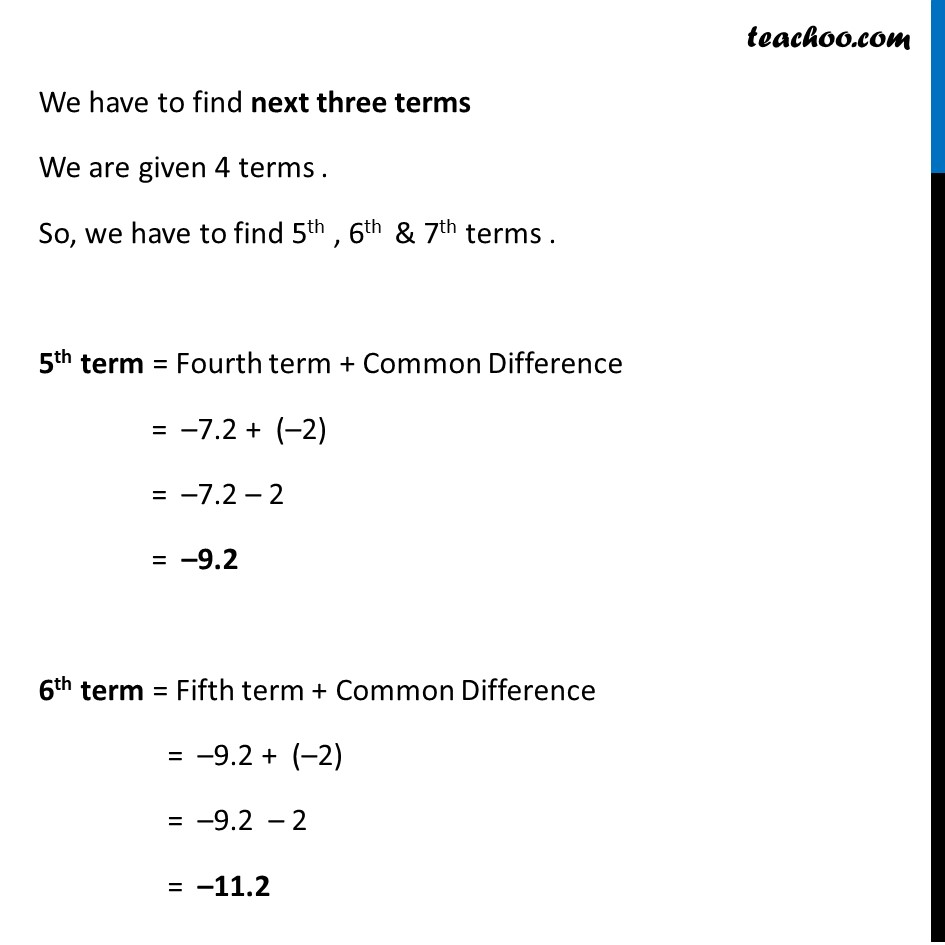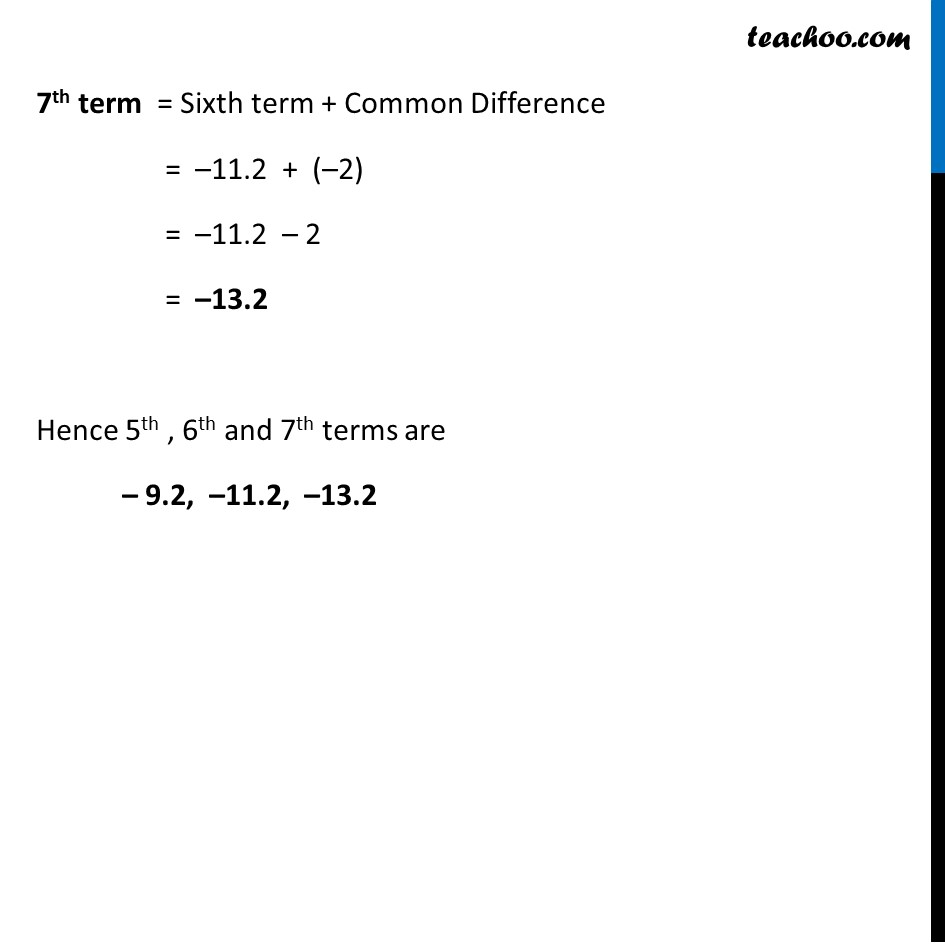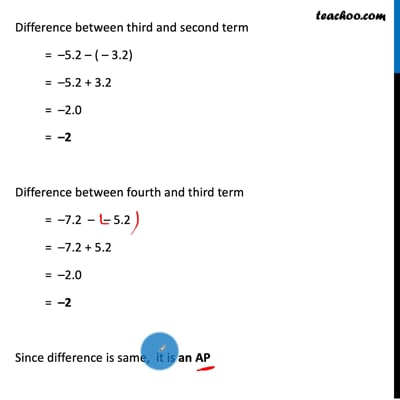This video is only available for Teachoo black users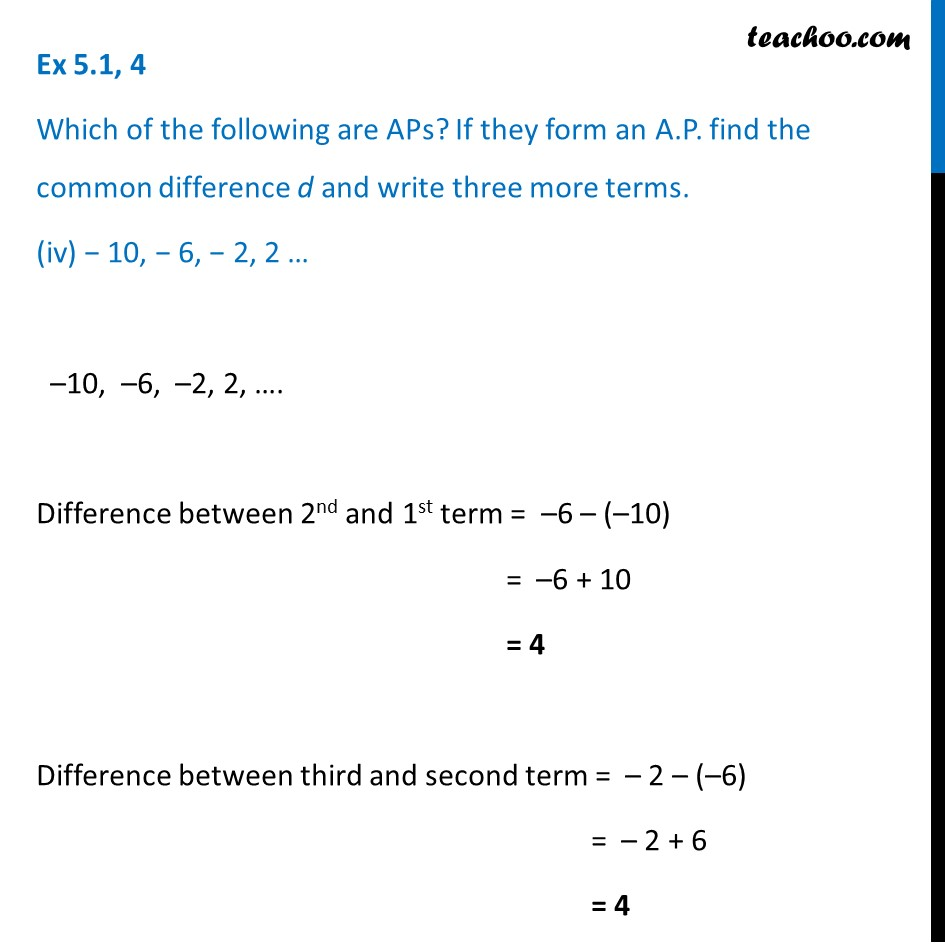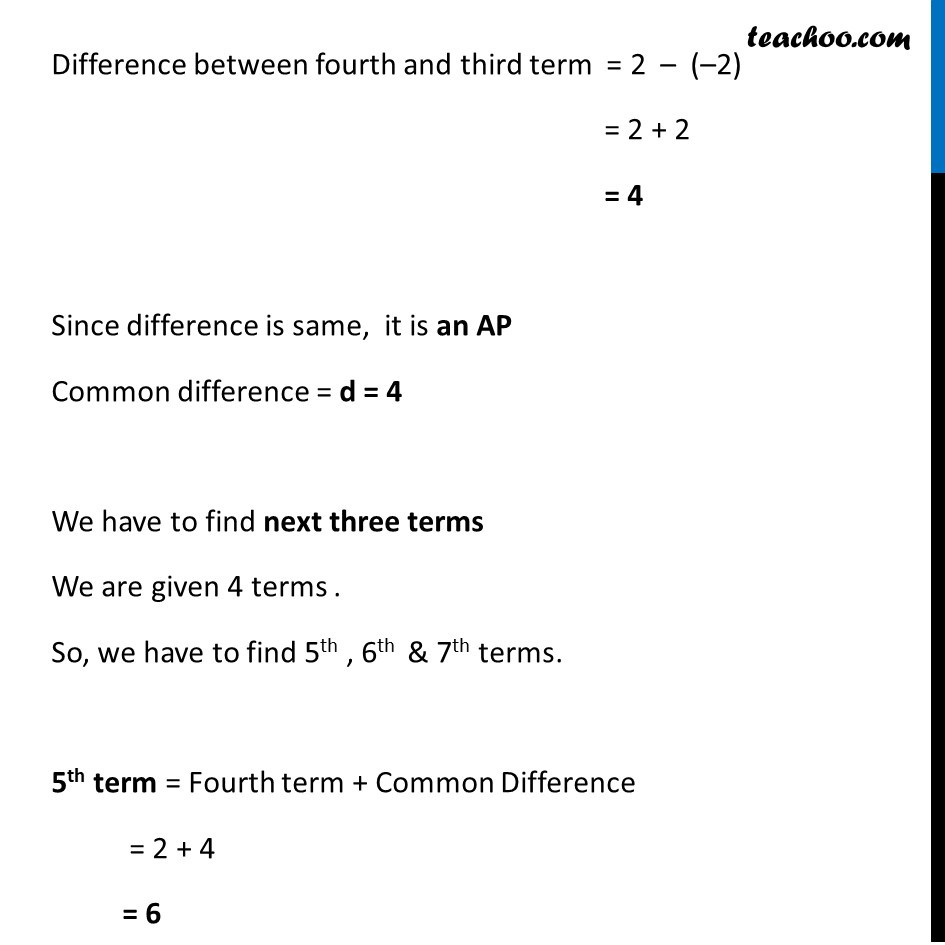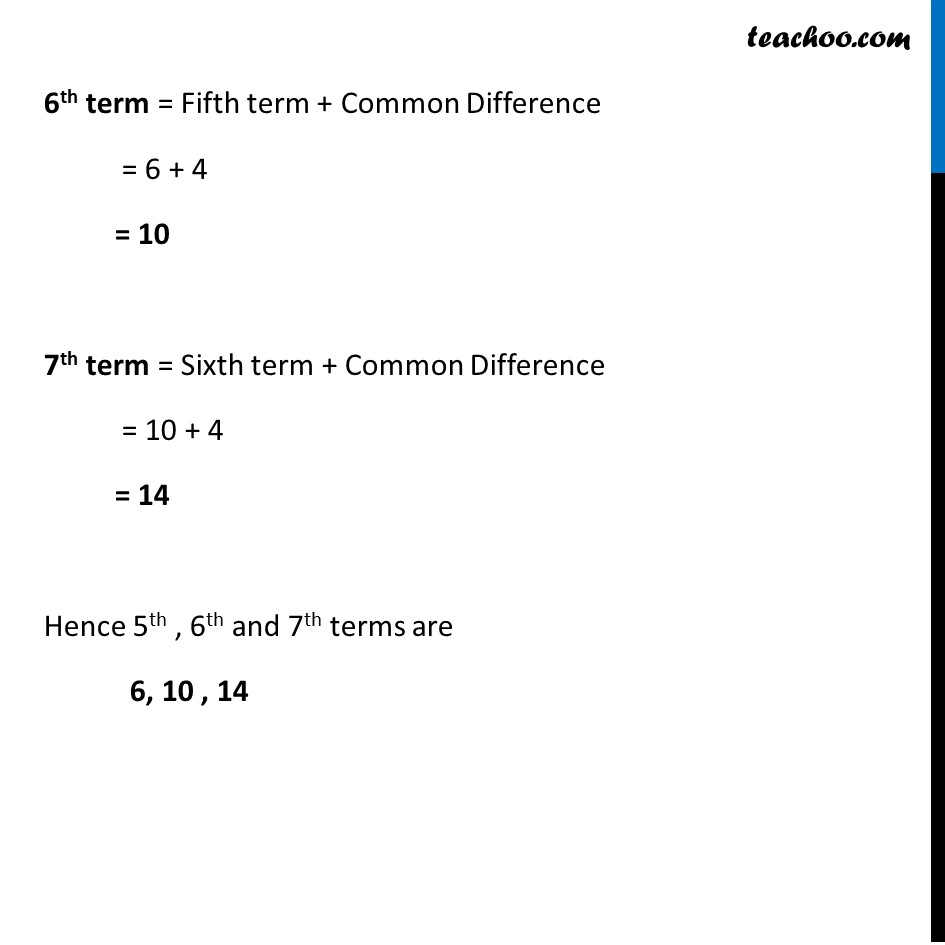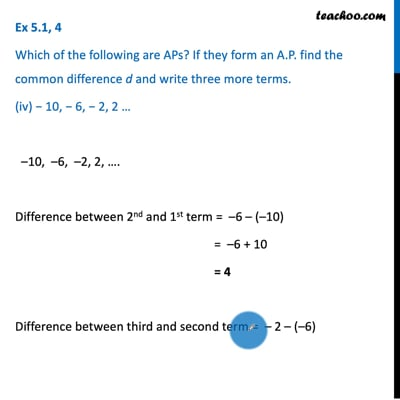This video is only available for Teachoo black users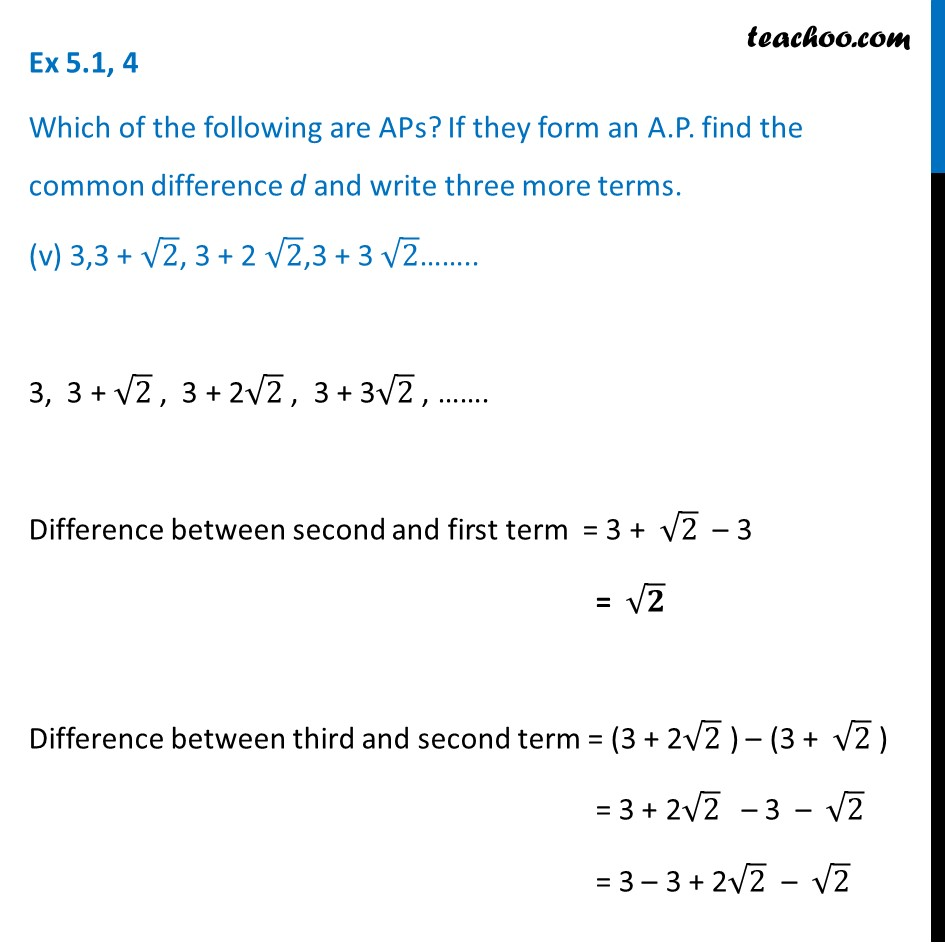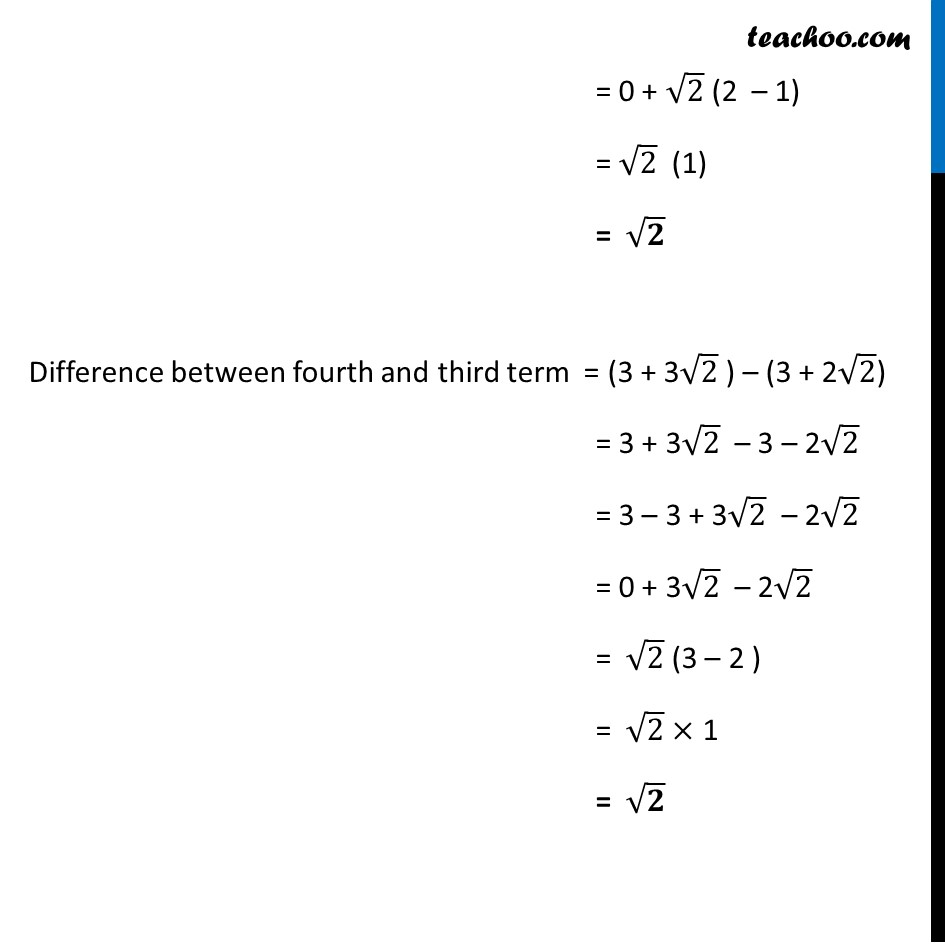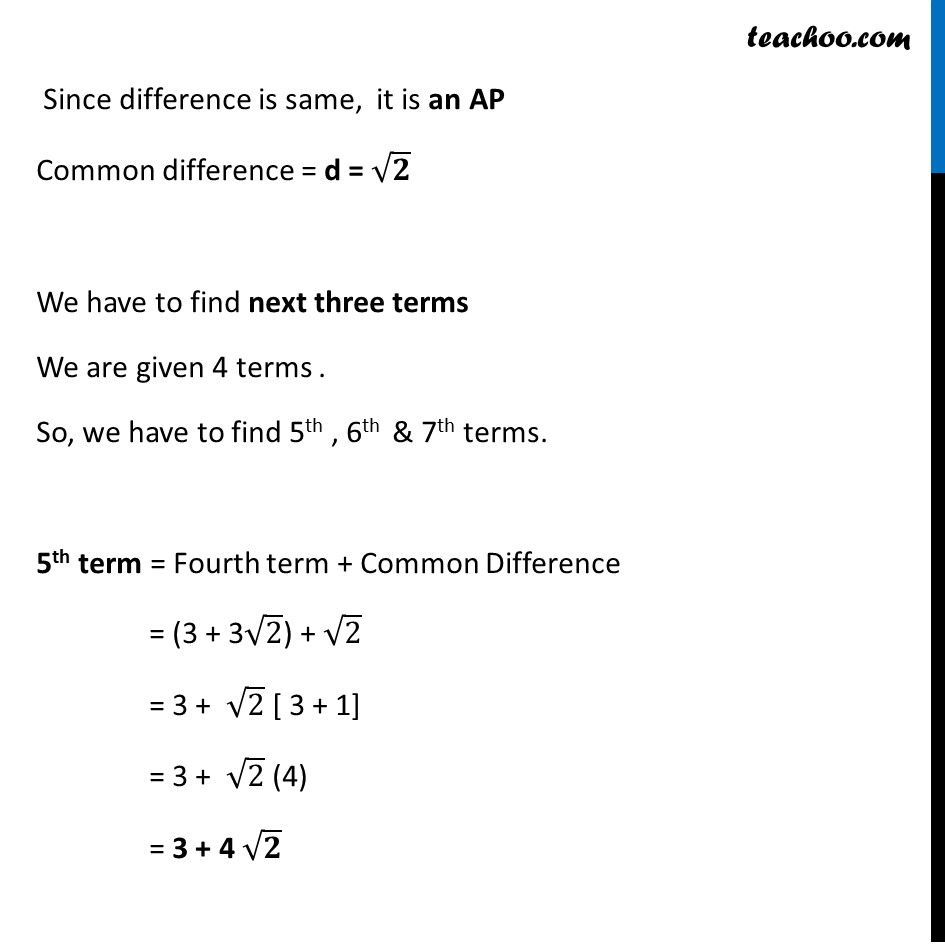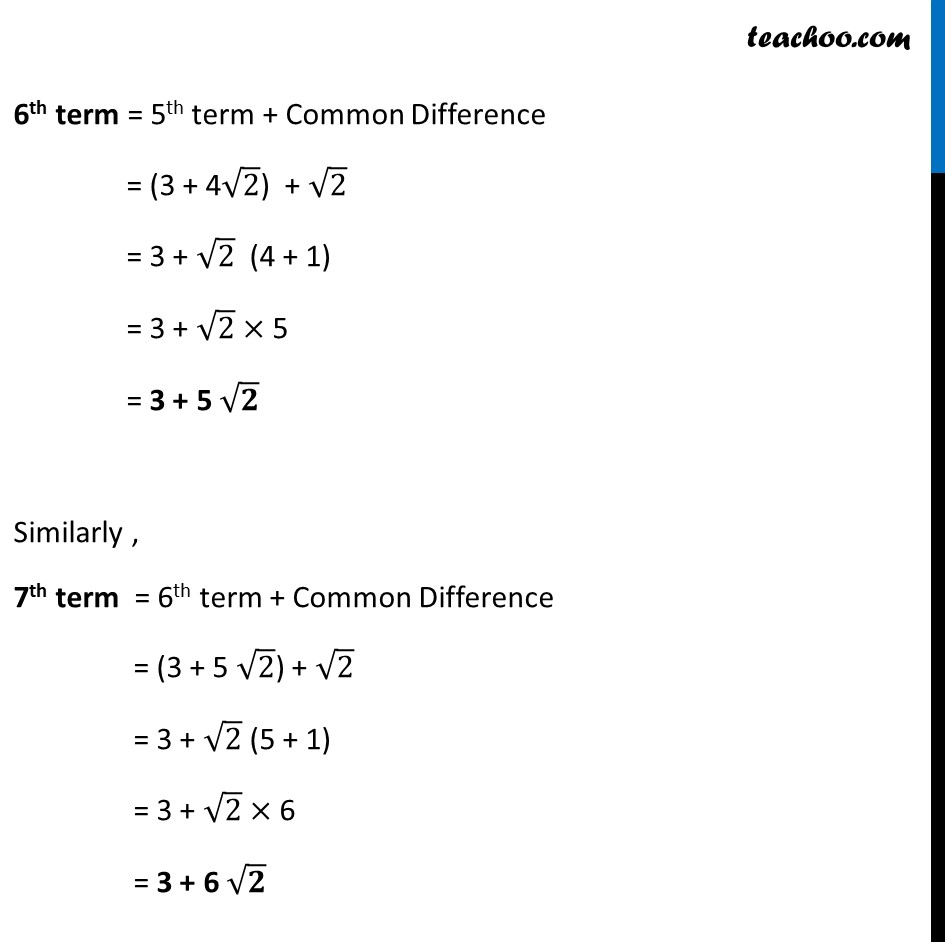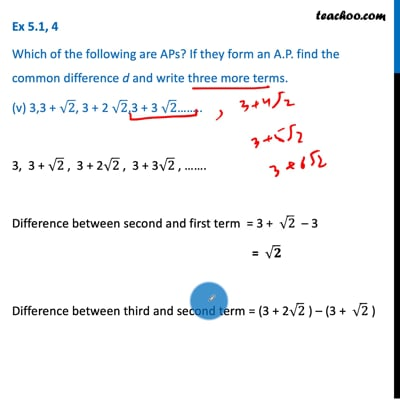This video is only available for Teachoo black users

### Transcript

Ex 5.1, 4 Which of the following are APs? If they form an A.P. Find the common difference d and write three more terms. (i) 2, 4, 8, 16 … 2, 4, 8, 16….. Difference of second and first term = 4 – 2 = 2 Difference of third and second term = 8 – 4 = 4 Since 2 ≠ 4 Difference is not same Hence, it is not an AP Ex 5.1, 4 Which of the following are APs? If they form an A.P. Find the common difference d and write three more terms. (ii) 2, 5/2, 3, 7/2….. 2, 5/2 , 3, 7/2 , ….. Difference between second and first term = 5/2 – 2 = (5 − 2 × 2)/2 = (5 − 4)/2 = 𝟏/𝟐 Difference between third and second term = 3 – 5/2 = (3 × 2 − 5)/2 = (3 × 2 − 5)/2 = (6 − 5)/2 = 𝟏/𝟐 Difference between fourth and third term = 7/2 – 3 = (7− 3 × 2)/2 = (7 − 6)/2 = 𝟏/𝟐 Since difference is same, it is an AP Common difference = d = 𝟏/𝟐 We have to find next three terms We are given 4 terms . So, we have to find 5th , 6th & 7th terms . 5th term = 4th term + common difference = 7/2 + 1/2 = (7 + 1)/2 = 8/2 = 4 6th term = 5th term + common difference = 4 + 1/2 = (4 × 2 + 1)/2 = 𝟗/𝟐 7th term = 6th term + common difference = 9/2 + 1/2 = (9 + 1)/2 = 10/2 = 5 Hence 5th , 6th and 7th terms are 4, 𝟗/𝟐 , 5 Ex 5.1, 4 Which of the following are APs? If they form an A.P. find the common difference d and write three more terms. (iii) − 1.2, − 3.2, − 5.2, − 7.2 … –1.2, –3.2, –5.2, –7.2 Difference between second and first term = –3.2 – (–1.2) = –3.2 + 1.2 = –2.0 = –2 Difference between third and second term = –5.2 – ( – 3.2) = –5.2 + 3.2 = –2.0 = –2 Difference between fourth and third term = –7.2 – – 5.2 = –7.2 + 5.2 = –2.0 = –2 Since difference is same, it is an AP Common difference = d = –2 We have to find next three terms We are given 4 terms . So, we have to find 5th , 6th & 7th terms . 5th term = Fourth term + Common Difference = –7.2 + (–2) = –7.2 – 2 = –9.2 6th term = Fifth term + Common Difference = –9.2 + (–2) = –9.2 – 2 = –11.2 7th term = Sixth term + Common Difference = –11.2 + (–2) = –11.2 – 2 = –13.2 Hence 5th , 6th and 7th terms are – 9.2, –11.2, –13.2 Ex 5.1, 4 Which of the following are APs? If they form an A.P. find the common difference d and write three more terms. (iv) − 10, − 6, − 2, 2 … –10, –6, –2, 2, …. Difference between 2nd and 1st term = –6 – (–10) = –6 + 10 = 4 Difference between third and second term = – 2 – (–6) = – 2 + 6 = 4 Difference between fourth and third term = 2 – (–2) = 2 + 2 = 4 Since difference is same, it is an AP Common difference = d = 4 We have to find next three terms We are given 4 terms . So, we have to find 5th , 6th & 7th terms. 5th term = Fourth term + Common Difference = 2 + 4 = 6 6th term = Fifth term + Common Difference = 6 + 4 = 10 7th term = Sixth term + Common Difference = 10 + 4 = 14 Hence 5th , 6th and 7th terms are 6, 10 , 14 Ex 5.1, 4 Which of the following are APs? If they form an A.P. find the common difference d and write three more terms. (v) 3,3 + √2, 3 + 2 √2,3 + 3 √2…….. 3, 3 + √2 , 3 + 2√2 , 3 + 3√2 , ……. Difference between second and first term = 3 + √2 – 3 = √𝟐 Difference between third and second term = (3 + 2√2 ) – (3 + √2 ) = 3 + 2√2 – 3 – √2 = 3 – 3 + 2√2 – √2 = 0 + √2 (2 – 1) = √2 (1) = √𝟐 Difference between fourth and third term = (3 + 3√2 ) – (3 + 2√2) = 3 + 3√2 – 3 – 2√2 = 3 – 3 + 3√2 – 2√2 = 0 + 3√2 – 2√2 = √2 (3 – 2 ) = √2 × 1 = √𝟐 Since difference is same, it is an AP Common difference = d = √𝟐 We have to find next three terms We are given 4 terms . So, we have to find 5th , 6th & 7th terms. 5th term = Fourth term + Common Difference = (3 + 3√2) + √2 = 3 + √2 [ 3 + 1] = 3 + √2 (4) = 3 + 4 √𝟐 6th term = 5th term + Common Difference = (3 + 4√2) + √2 = 3 + √2 (4 + 1) = 3 + √2 × 5 = 3 + 5 √𝟐 Similarly , 7th term = 6th term + Common Difference = (3 + 5 √2) + √2 = 3 + √2 (5 + 1) = 3 + √2 × 6 = 3 + 6 √𝟐